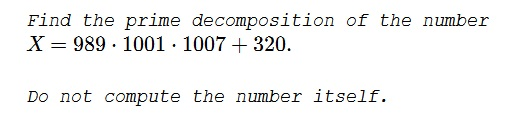# Looking for the Prime Decomposition

### Problem### Solution 1

Let $Y=991.$ Then

\begin{align} X&=(Y-2)(Y+10)(Y+16)+320\\ &=Y^3+24Y^2+108Y-320+320\\ &=Y(Y^2+24Y+108)=Y(Y+6)(Y+18)\\ &=991\cdot 997\cdot 1009. \end{align}

All three factors are prime. Suffice it to check that they are not divisible by $3,$ $5,$ $7,$ $11,$ $13,$ $17,$ $19,$ $23,$ $29,$ $31$ which are the only prime numbers less than $\sqrt{1009}$ - the only possible factors.

$3,5,11$ are discharged right away as they fail the divisibility tests.

For $7$ we can subtract $980=700+7\cdot 40$ from the three numbers to get $11,17,29,$ none of which is divisible by $7.$

For $13,$ similarly, we subtract $910=13\cdot 70$ to obtain $1,7,19$ none of which is divisible by $13.$

For $17,$ $19,$ $23,$ $29,$ $31$ the convenient multiples are $1020=17\cdot 60,$ $950=19\cdot 50,$ $920=23\cdot 40,$ $870=29\cdot 30,$ and $930=31\cdot 30.$

### Remark

Solution comes as a mystery but in the afterthought we observe that its based on the decomposition $320=2\cdot 10\cdot 16.$ Since that's not the only possibility, we may try a few others. Computers and software make that easy. But the bottom line, all the decompositions I tried came up with irrational roots.

It appears a non-trivial question when and whether two polynomials with integer roots have a constant integer difference. At the reference below it was observed that pairs of such polynomials were found for the degrees $4,$ $5,$ and $6.$

### Solution 2

Let $Y=995.$ Then

\begin{align}(Y-6)(Y+6)(Y+12)+320&=(Y-4)(Y+2)(Y+14)\\ &=991\cdot 997\cdot 1009. \end{align}

### Solution 3

One way is to hope we get lucky and look for $x$ such that $(989-x)(1001-x)(1007-x) = -320.$ Fortunately $x=991$ works, as we get $-2\cdot 10\cdot 16.$ Hence we have $(x-2)(x+10)(x+16)-320 = x^3+24x^2+108x = x(x+6)(x+18),$ so we get $991\cdot 997\cdot 1009.$ Checking that these are prime is straightforward.

### Acknowledgment

This is a problem from the book Leningrad Mathematical Olympiad: 1987-1991 by D. Fomin and A. Kirichenko, (MathPro Press, 1994, #42-1987)

Solution 2 is by Leo Giugiuc; Solution 3 is by Christopher D. Long.